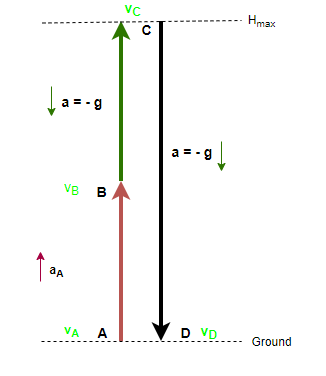# Problem: A model rocket has a constant upward acceleration of 40.0 m/s2 while its engine is running. The rocket is fired vertically, and the engine runs for 2.50 s before it uses up the fuel. After the engine stops, the rocket is in free fall. The motion of the rocket is purely up and down.(a) What is the maximum height that the rocket reaches?(b) What will be the speed of the rocket just before it hits the ground?

###### FREE Expert Solution

We are asked to calculate the maximum height reached by the rocket and its speed just before hitting the ground.

This is a kinematics problem involving a vertical launch of a rocket with motion in multiple parts.

The steps followed for this kind of problem are simple and straightforward:

1. Draw diagram, axes.
2. Develop equations to describe the various intervals.
3. Solve each of the target variables.

Step 1:  Draw diagram, axes.The rocket is launched vertically upwards with a constant upward acceleration (aA), which lasts 2.50 s. At the instant when the engine stops, the rocket has an upward velocity, which is reduced by gravitational acceleration to zero at the maximum height. After the maximum height, the rocket remains in free fall until it hits the ground. Therefore, our problem has three intervals of motion.

We are going to use a standard coordinate system where positive y-axis points upwards. This means that velocity of the rocket upwards is positive and velocity downward is negative.

90% (271 ratings)###### Problem Details

A model rocket has a constant upward acceleration of 40.0 m/s2 while its engine is running. The rocket is fired vertically, and the engine runs for 2.50 s before it uses up the fuel. After the engine stops, the rocket is in free fall. The motion of the rocket is purely up and down.
(a) What is the maximum height that the rocket reaches?
(b) What will be the speed of the rocket just before it hits the ground?